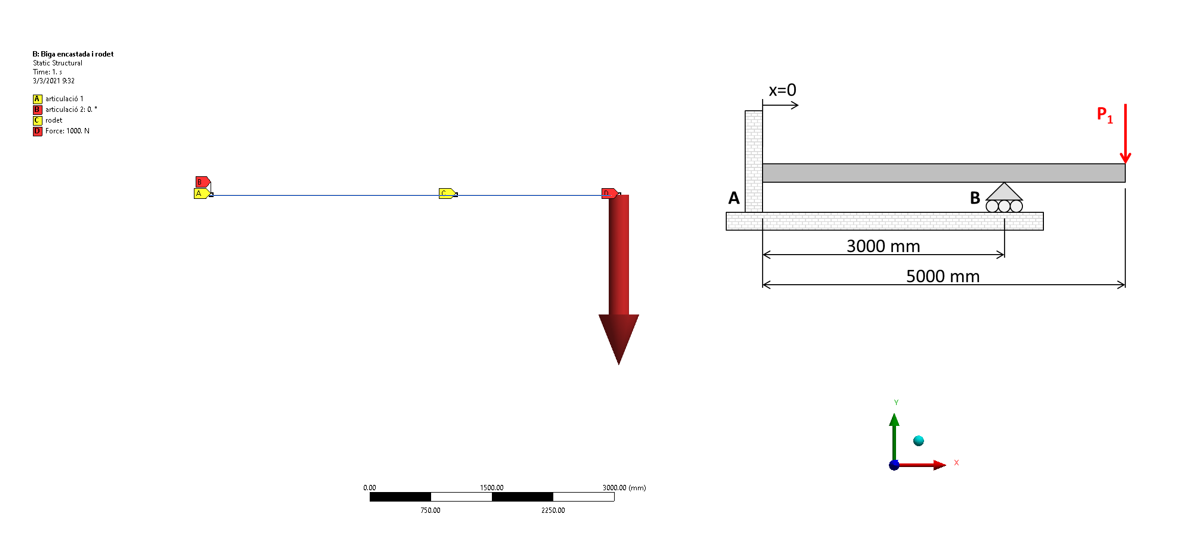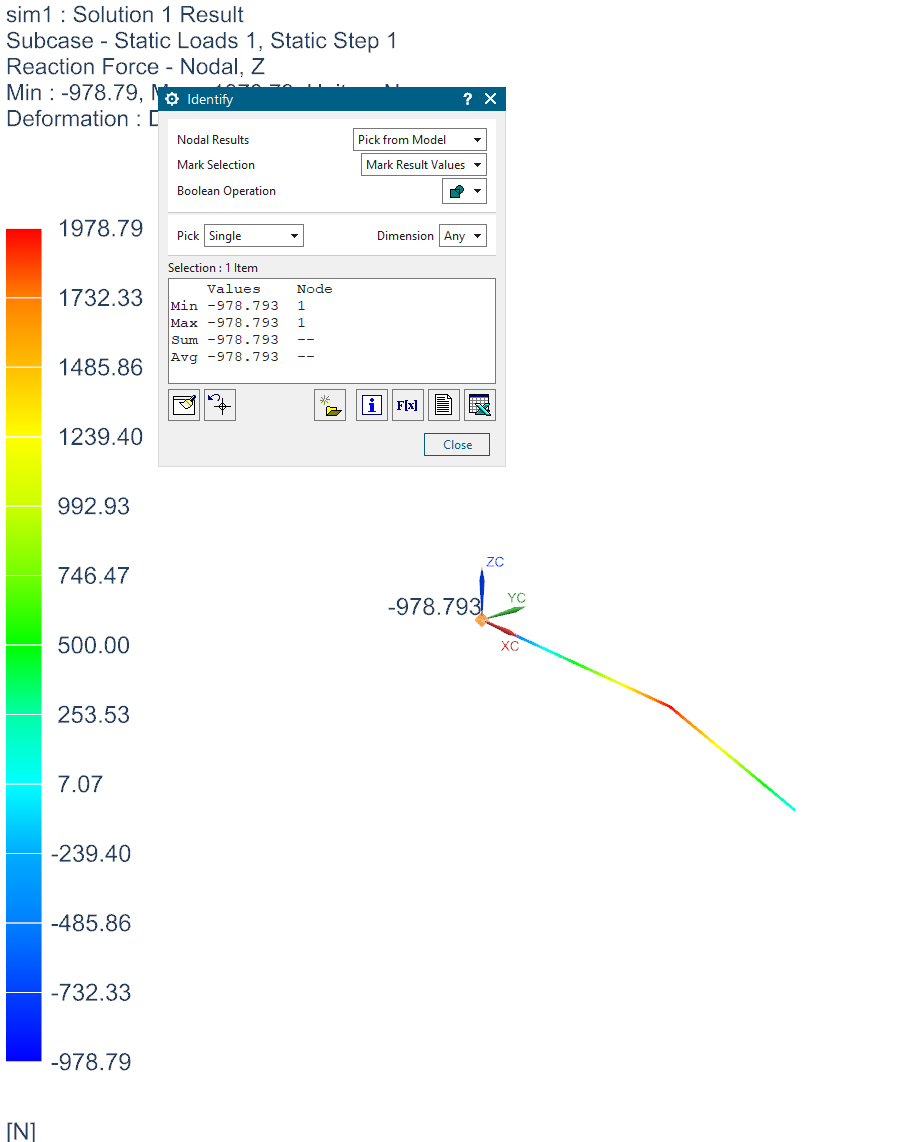## General Mechanical

•jordimarce
Subscriber
I am solving a cantilever beam with more constraints as an statically indeterminate problem (hyperstatic beam) and the reactions in the cantilever are mathematically wrong. Sould be 1000 N and I get 969.86 irrespective of the mesh and other input parameters I checked carefully. nI can solve it by hand and I also solved the same problem in another software so, I am pretty sure that this is a error from ANSYS 2019 R3. Is this a known bug? If not, is it somenthing I am missing?n
•Kai
Ansys Employee
I can think of 2 things that may contribute to the difference. Ansys considers geometry nonlinearity (I assume you turned on large deflection) and uses Timoshenko beam theory for beam element. Hand calculation doesn't account for geometry nonlinearity and are typically based on Euler?Bernoulli beam theory.n
•jordimarce
Subscriber
Large deflection are OFF so, I am solving a simple linear problemn
•jordimarce
Subscriber
Any more idea? n
•BenjaminStarling
Subscriber
How are you applying the load to the structure? Is this using Mechanical or Mechanical APDL? If in Mechanical, the default application of loads uses surface elements, which can lead to some rounding errors. We would need more information about your project to assist further. Screenshots of your model and loads is a good place to start.n
•jordimarce
Subscriber
It Is a simple cantilever beam model with a rectangular section. Fully fixed in the left side (roations zero and displacements zero or fixed, which has the same results) and an articulation in the middle (only fixed displacements in y). A force in the right side. The horitzontal reaction in the fixed left side should be (theoretically) 1000 N (down) and ANSYS give me 969.99 N (down). Othe resullts such as the moment and the maximum displacement are not the same than the theoretical. I checked the model in other softwares and I got the same results than the teorethical. Therefore, the problem I have is in the ANSYS model.nn
•BenjaminStarling
Subscriber
nI ran the same model and got the result I expected, 990N, compared to your 970N, but the actual reaction force is 1000N. (I am not sure why we get such differences in rounding)nMy guess is that other software you used are either beam analysis packages, and not full FE packages.nTo understand this result, you need to understand that the FE method does not solve for force, and force is preserved only on average, across the domain. To check this out for yourself, under output controls, turn nodal forces on, then resolve. Plot the user defined result, enfovectors. You will see the 1000N reaction force, but also that each element has it's own reaction force, and at nodes shared by two elements, there are two results, both different.n
•jordimarce
Subscriber
Hi Array : I got the same result 970 N.nYes, I run a beam model in a beam analysis package, but the results should be the same irrespective of the method. Also, I am aware of the mathematical formulation of FEM and that the resolution of the matrix is in the nodes. So, I expected the reaction in the node to be the same than I manually solved. I attach the model, maybe you can have a look and see if you and me are doing the same resolution because maybe this is just what is wrong in my model. Thank you!nnArrayPD:Just to say that:This is one example we run at class with the strudents, so I want to understand what's going wrong in my FEA model n
•BenjaminStarling
Subscriber
Again, there is nothing wrong. This is to be expected with FEM. The reaction force at the support on the right is 1000 N, the reaction on the left is 1000 N minus some error due to the coupling of strain to force. Beam packages usually are not solving partial derivatives, just a bunch of simultaneous equations that have pre-baked stiffness equations, this is why they yield perfect force results, and why they generally only do one type of analysis (piping, structural beams.. etc.) It is also why they generally cannot do transient analysis, as the mass term is not coupled, but can do Modal Superposition.nTwo things you can do to test this result.nChange the cross section to rectangular solid. Use 1 mm x 1000 mm, then run another analysis where it is 1000 mm x 1 mm.nSet the poissons ratio to 0. (or vary it numerous times and see the change in reaction force)nI ran the same simulation in Simcenter (NX Nastran) and get the same result for a 500x500mm cross section (978.793 (NX) vs. 979.01 (Ansys))nn
•jordimarce
Subscriber
Ok. Thanks for the time and the explanation!n